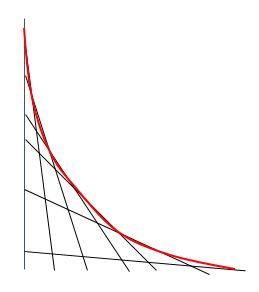# Tangential Network 1

Calculus Level 5Let $n$ be a real number; define the curve $C_n$ by the equation

$x^n + y^n = 1.\ \ \ \ x, y \geq 0$

For every point $x, y$ on $C_n$ there is a tangent line. This tangent line intersects the coordinate axes in points $(\xi,0)$ and $(0,\eta)$, respectively.

There exists one curve $C_n$ for which the value of $\xi + \eta$ is the same for all these tangent lines. How much is $n$?

×

Problem Loading...

Note Loading...

Set Loading...